Updating search results...

# 121 Results

View
Selected filters:
• word-problemsConditional Remix & Share Permitted
CC BY-NC
Rating
0.0 stars

Students review the standard long-division algorithm and discuss the different ways the answer to a whole-number division problem can be expressed (as a whole number plus a remainder, as a mixed number, or as a decimal).Students solve a series of real-world problems that require the same whole number division operation, but have different answers because of how the remainder is interpreted.Key ConceptsStudents have been dividing multidigit whole numbers since Grade 4. By the end of Grade 6, they are expected to be fluent with the standard long-division algorithm. In this lesson, this algorithm is reviewed along with the various ways of expressing the answer to a long division problem. Students will have more opportunities to practice the algorithm in the Exercises.Goals and Learning ObjectivesReview and practice the standard long-division algorithm.Answer a real-world word problem that involves division in a way that makes sense in the context of the problem.

Subject:
Mathematics
Material Type:
Lesson Plan
Author:
Angela Vanderbloom
07/28/2018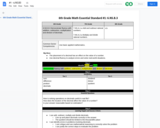Conditional Remix & Share Permitted
CC BY-NC-SA
Rating
0.0 stars

The placement of a decimal has an effect on the value of a number.
Use decimal fluency to analyze errors and solve real-world situations.

Subject:
Mathematics
Material Type:
Activity/Lab
Author:
Liberty Public Schools
04/09/2021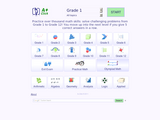Conditional Remix & Share Permitted
CC BY-NC-SA
Rating
0.0 stars

A+ Click is an interactive collection of more than 3700 math problems and answers for K-1 K-12 school program. It defines the personal level of math knowledge. You move up into the next level if you give 5 correct answers in a row. Practice makes perfect.

Subject:
Algebra
Geometry
Mathematics
Material Type:
Activity/Lab
Assessment
Game
Lecture Notes
Provider:
NSDL Staff
Provider Set:
Mathematics Gateways and Resources
Author:
Igor Kokcharov
02/16/2011Conditional Remix & Share Permitted
CC BY-NC-SA
Rating
0.0 stars

This is an interactive assessment and video designed to teach Adding and Subtracting (Numbers up to 20) - Word Problems to first graders.

Subject:
Mathematics
Material Type:
Assessment
Interactive
Lecture
Provider:
CK-12 Foundation
Provider Set:
CK-12 Elementary Math
02/27/2015Only Sharing Permitted
CC BY-NC-ND
Rating
0.0 stars

National Board Certified teacher Marlo Warburton introduces a fresh approach to teaching the notoriously difficult "mixture" word problems for Algebra I. Using an in-class demonstration and incorporating basic physics principles of weight and distance, Ms. Warburton helps her students visualize, solve -- and even enjoy! -- these challenging problems.

Subject:
Algebra
Education
Mathematics
Material Type:
Lesson Plan
Teaching/Learning Strategy
Provider:
Teaching Channel
Provider Set:
Teaching Channel
11/01/2012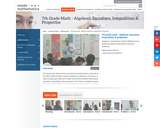Rating
0.0 stars

This lesson is about trying to get students to make connections between ideas about equations, inequalities, and expressions. The lesson is designed to give students opportunities to use mathematical vocabulary for a purpose to describe, discuss, and work with these symbol strings.The idea is for students to start gathering global information by looking at the whole number string rather than thinking only about individual procedures or steps. Hopefully students will begin to see the symbol strings as mathematical objects with their own unique set of attributes. (7th Grade Math)

Subject:
Algebra
Mathematics
Numbers and Operations
Material Type:
Activity/Lab
Lecture
Lesson Plan
Teaching/Learning Strategy
Provider:
Noyce Foundation
Provider Set:
Inside Mathematics
Author:
Disston, Jacob
11/30/2011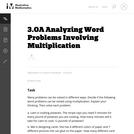Unrestricted Use
CC BY
Rating
0.0 stars

In this task, the students are not asked to find an answer, but are asked to analyze word problems and explain their thinking. In the process, they are faced with varying ways of thinking about multiplication.

Subject:
Mathematics
Numbers and Operations
Material Type:
Activity/Lab
Provider:
Illustrative Mathematics
Provider Set:
Illustrative Mathematics
Author:
Illustrative Mathematics
05/01/2012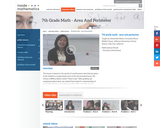Rating
0.0 stars

This lesson is based on the results of a performance task in which we realized that students' understanding of area and perimeter was mostly procedural. Therefore the purpose of this re-engagement lesson was to address student misconceptions and deepen student understanding of area and perimeter. The standards addressed in this lesson involve finding perimeter and area of various shapes, finding the perimeter when given a fixed area, and using a formula in a practical context. Challenges for our students included decoding the language in the problem and proving their thinking. (7th Grade Math)

Subject:
Mathematics
Material Type:
Activity/Lab
Lecture
Lesson Plan
Teaching/Learning Strategy
Provider:
Noyce Foundation
Provider Set:
Inside Mathematics
Author:
Villarin, Antoinette
11/30/2011Unrestricted Use
CC BY
Rating
0.0 stars

This series of word problems requires students to apply the concepts of factors and common factors in a context.

Subject:
Mathematics
Material Type:
Activity/Lab
Provider:
Illustrative Mathematics
Provider Set:
Illustrative Mathematics
Author:
Illustrative Mathematics
05/01/2012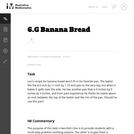Unrestricted Use
CC BY
Rating
0.0 stars

The purpose of this task is to provide students with a multi-step problem involving volume and to give them a chance to discuss the difference between exact calculations and their meaning in a context.

Subject:
Geometry
Mathematics
Material Type:
Activity/Lab
Provider:
Illustrative Mathematics
Provider Set:
Illustrative Mathematics
Author:
Illustrative Mathematics
05/01/2012Some Rights Reserved
Rating
0.0 stars

Try this fun problem! In any group of six people, what is the probability that everyone was born in different months?

Subject:
Education
Mathematics
Material Type:
Diagram/Illustration
Lecture Notes
Provider:
SMARTR
Provider Set:
SMARTR: Virtual Learning Experiences for Youth
Author:
KnowNet Construction, Inc.
11/06/2004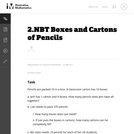Unrestricted Use
CC BY
Rating
0.0 stars

This instructional task requires students to figure out word problems that require thinking in base 10.

Subject:
Mathematics
Numbers and Operations
Material Type:
Activity/Lab
Provider:
Illustrative Mathematics
Provider Set:
Illustrative Mathematics
Author:
Illustrative Mathematics
05/01/2012Unrestricted Use
CC BY
Rating
0.0 stars

MATH&148 is a calculus course for business students. It is designed for students who want a brief course in calculus. Topics include differential and integral calculus of elementary functions. Problems emphasize business and social science applications. Translating words into mathematics and solving word problems are emphasized over algebra. Applications are mainly business oriented (e.g. cost, revenue, and profit). Mathematical theory and complex algebraic manipulations are not mainstays of this course, which is designed to be less rigorous than the calculus sequence for scientists and engineers. Topics are presented according to the rule of four: geometrically, numerically, analytically, and verbally. That is, symbolic manipulation must be balanced with graphical interpretation, numerical examples, and writing. Trigonometry is not part of the course.

Subject:
Calculus
Mathematics
Material Type:
Assessment
Full Course
Syllabus
Textbook
Provider:
Washington State Board for Community & Technical Colleges
Provider Set:
Open Course Library
05/03/2013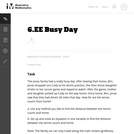Unrestricted Use
CC BY
Rating
0.0 stars

This task provides a good entry point for students into representing quantities in contexts with variables and expressions and building equations that reflect the relationships presented in the context.

Subject:
Mathematics
Material Type:
Activity/Lab
Provider:
Illustrative Mathematics
Provider Set:
Illustrative Mathematics
Author:
Illustrative Mathematics
08/15/2012Unrestricted Use
CC BY
Rating
0.0 stars

There are two aspects to fluency with division of multi-digit numbers: knowing when it should be applied, and knowing how to compute it. While this task is very straightforward, it represents the kind of problem that sixth graders should be able to recognize and solve relatively quickly.

Subject:
Mathematics
Material Type:
Activity/Lab
Provider:
Illustrative Mathematics
Provider Set:
Illustrative Mathematics
Author:
Illustrative Mathematics